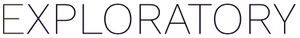How can I calculate the mean value between two columns?

#1

You can use ‘Mutate (Create Calculation)’ and have something like the below.

(<columnA> + <column B> ) / 2
#2

The calculation won’t be correct if there is a missing value in one of the columns

You can use ifelse() function.

ifelse(is.na(<colA>) or is.na(<colB>), NA, (<columnA> + <column B> ) / 2)